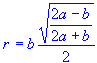# Total area of circles infinitely inscribed in isosceles triangle

1. The base of the yellow triangle has length 8 inches; its height is 10 inches. Each of the circles is tangent to each edge and each other circle that it touches. There are infinitely many circles. The radius of the largest of them is __________ and the total area of all the circles is ___________.

There's a picture with the largest circle centered at the base of the triangle, and continously smaller circles until the peak of the triangle.

n/a

## The Attempt at a Solution

I figured out the radius of the largets circle by using this equation:and got 2.708131846.

The problem I'm having is determining the correct infinite series to figure out the area of all the triangles. I know the area of each circle is pi*r^2, but the radius of each circle is dependent on the sides of the triangle, which change for each circle.

LCKurtz
Homework Helper
Gold Member
I don't see a picture but I assume from your calculations that the triangle is isosceles and the circles are stacked vertically. If I am not correct about that, ignore the rest of this post.
Call the base b and the height a for the triangle, and lets call r the radius of the largest circle.

Draw a line across the top of the first circle parallel to the base making a little sub-triangle which is similar to the original triangle for the next circle. The height of the little triangle is a-2r so the little triangle is proportional to the big triangle by a factor of

[tex]\lambda = \frac{a-2r} a[/itex]

Area goes by the square of the linear dimensions, so if the area of your first circle is A, then the area of the next is $\lambda^2A$ and the next is $\lambda^4A$ and so on. That should help.

Thank you so much - that was very helpful.